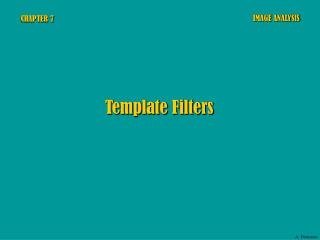# CHAPTER 7 - PowerPoint PPT PresentationDownload PresentationCHAPTER 7

CHAPTER 7
Download Presentation## CHAPTER 7

- - - - - - - - - - - - - - - - - - - - - - - - - - - E N D - - - - - - - - - - - - - - - - - - - - - - - - - - -
##### Presentation Transcript

1. IMAGE ANALYSIS CHAPTER 7 Template Filters A. Dermanis

2. Moving templates for image filtering gij = fi–1,j–1h–1,–1 + fi–1,jh–1,0 + fi–1,j+1h–1,1 + + fi,j–1h0,–1 + fi,jh0,0 + fi,j+1h0,1 + + fi+1,j–1h1,–1 + fi+1,jh1,0 + fi+1,j+1h1,1 The discrete convolution process in template filtering A. Dermanis

3. Typical template dimensions Non-square templates viewed as special cases of square ones A. Dermanis

4.  linear gij = hk–i,m–jfkm k m hi,j;k,m = hk–i,m–j position-invariant  gij = hi,j;k,mfkm k m i+p j+p  gij = hi,j;k,mfkm localized k=i–p m=j–p Template filters = Localized position-invariant lineartransformations of an image Using a (p+1)(p+1) template A. Dermanis

5. k = k–i m = m– j i+p j+p p p p p    gij = hk–i,m–jfkm gij = hk,mfi+k,j+m g00 = hk,mfk,m k=–p m=–p k=i–p m=j–p k=–p m=–p Template filters = Localized position-invariant lineartransformations of an image Combination of all properties renamed (i=0, j=0, k=k, m=m) A. Dermanis

6. i–1 i i+1 j–1 j j+1 p p  g00 = hk,mfk,m k=–p m=–p Template filters = Localized position-invariant lineartransformations of an image renamed hij fij g00 = h–1,–1f–1,–1 + h –1,0f–1,+1 + h –1,1f–1,+1 + + h0,–1f0,–1 + h0,0f0,0 + h0,+1f0,+1 + + h+1,–1f+1,–1 + h+1,0f+1,0 + h+1,+1f+1,+1 A. Dermanis

7. fkm = C fkm = C 1 1 25 9 homogeneous (low frequency) areas preserve their value homogeneous areas are set to zero high values emphasize high frequencies p p p p p p p p     g00 = hk,mC = 0 g00 = hk,mC = C hk,m= 0 hk,m= 1 Examples Examples k=–p m=–p k=–p m=–p k=–p m=–p k=–p m=–p Low-pass filters High-pass filters A. Dermanis

8. An example of low pass filters: The original band 3 of a TM image is undergoing low pass filtering by moving mean templates with dimensions 33 and 55 Original Moving mean 33 Moving mean 55 A. Dermanis

9. An example of a high pass filter: The original image is undergoing high pass filtering with a 33 template, which enhances edges, best viewed as black lines in its negative Original high pass filtering 33 high pass filtering 33 (negative) A. Dermanis

10. hkmfkm k,m Templatesexpressing linear operators Local interpolation and templateformulation fkm f(x, y) interpolation A evaluation g(x, y) gij g(0,0) A. Dermanis

11. 22 A =  = + x2y2 The Laplacian operator Examples of Laplacian filters with varying template sizes Original (TM band 4) Laplacian 99 Laplacian 1313 Laplacian 1717 A. Dermanis

12. Examples of Laplacian filters with varying template sizes Original (TM band 4) Laplacian 55 Original + Laplacian 55 A. Dermanis

13. X2+Y2 X2+Y2 The Roberts and Sobel filters for edge detection Sobel filter Roberts filter X Y X Y Roberts Original (TM band 4) Sobel A. Dermanis